# Electrical Principles

## Electronic Theory

Before one can understand the field of EET, one must first be comfortable with the foundations upon which it is built. This lesson seeks to provide students with that foundation. We shall begin by looking at the basic building blocks of matter.

### Atomic Structure

All matter in nature is composed of compounds of elements or pure elements. These in turn have molecular structure which in turn contain atoms. Atoms are the fundamental particles of the chemical elements.

Atoms also have a structure. To examine it we shall consider the simplest element, Hydrogen.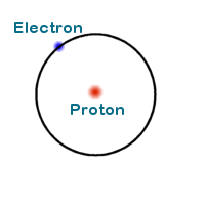Atom of Hydrogen

The center of the atom is called the nucleus. In the case of hydrogen the nucleus is a proton. Revolving around the nucleus is an electron.

The electron is a fundamental subatomic particle that has a negative charge and negligible mass. The proton which resides in the nucleus has a positive charge and a mass that is 1836 times the mass of an electron. The magnitudes of the proton and electron charges are identical.

There is one more subatomic particle that exists. It is found in the nucleus, has no charge, has a mass of 1839 electrons and is called a neutron.

Different elements have different numbers of protons. Hydrogen has 1 proton, helium has 2 protons, lithium - 3, neon - 10, iron - 26, and uranium - 92. Since atoms are electrically uncharged they have the same number of electrons as protons. So, for example, an atom of helium has 2 electrons.

So, elements are identified by their proton number which is also called atomic number. Thus the atomic number of iron is 26.

Let us look at the helium atom.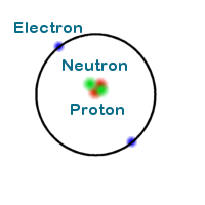Atom of Helium

The helium atom has 2 electrons, 2 protons and 2 neutrons (shown in green.) Since the mass of an atom is determined by the nucleus one can plainly see that the atomic mass of helium is four time the atomic mass of hydrogen.

Different atoms of elements may contain different numbers of neutrons. Atoms with the same atomic number but different neutron number are called isotopes. For instance, there are 3 isotopes of hydrogen, hydrogen with no neutrons, deuterium with 1 neutron and tritium with 2. In nature there are 2 isotopes of carbon,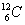and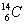which have 6 and 8 neutrons respectively.

In the above paragraph we are introduced to the nomenclature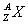,

where A is the mass number (Sum of protons and neutrons)
Z is the Atomic Number (number of protons), and
X is the Chemical Symbol for the element

#### Electronic Structure

Now let us now focus our attention on electronic structure by fist examining the lithium atom.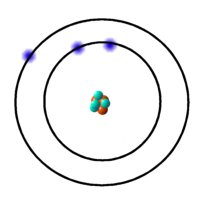Isotope of Lithium

The lithium isotope shown has 4 neutrons, 3 protons and 3 electrons. Here we see that the electrons revolve around the nucleus in different shells.

The shell closest to the nucleus called the K shell can hold 2 electrons. The next shell, moving outwards from the nucleus, is called the L shell and it holds up to 8 electrons. Next come M which also holds 8 (up to 18 in case of the transition elements). Then O etc. To exemplify, consider nitrogen which has an atomic number of 7. Clearly an atom of nitrogen has 7 electrons. We fill the inner shells first - 2 electrons in K shell. That leaves 5 electrons which fill the L shell.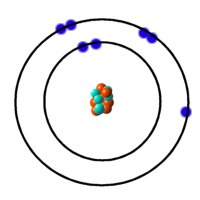Nitrogen Atom

The distance of the shells from the nucleus increases in the order K, L, M, N ... where K is closest. The electrons in the shells closest to the nucleus are more greatly influenced by the positive charge of the nucleus. Electrons in the outer shells experience a weaker attraction to the nucleus because of their greater distance from the nucleus and because of the shielding effect of electrons in inner shells.

#### Ionization

As mentioned in the previous paragraph electrons in outer shells  are more loosely bound to the atom than inner shell electrons. When given sufficient energy these electrons may become free of the influence of the atom. When this happen free electrons are released. The atom being left with a net positive charge is called an ion. When metals are heated in a vacuum electrons are emitted (cathode rays). This phenomenon is known as thermionic emission.

#### Structure of Matter

In this universe all things physical is matter. That which is not matter is energy. They are separate and distinct in the macroscopic world yet in the subatomic world we see that matter and energy are inter-convertible.

Element
An element is composed of atoms of one atomic number.

Compound
A compound is made from different elements. It thus contains atoms of different atomic numbers.
Molecule
A molecule is formed by the union of two or more atoms.

Molecules form to fill the empty spaces in atomic shells. The bonding of atoms as molecules is due to the sharing of electrons between atoms. For instance, hydrogen exists naturally as a molecule comprising 2 hydrogen atoms.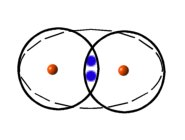Hydrogen Molecule

By sharing electrons each atom has it's shell filled.

Water is a compound whose molecular structure comprises of 2 hydrogen atoms and 1 oxygen atom. (Atomic oxygen has 6 electrons in its L shell)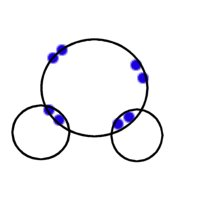Bonding in Water molecule

Much larger structures exist in nature. In the case of metals the entire macro-structure may be a lattice of atoms. The electrons of the outermost shells are so loosely bound to the atom that they are free to drift about from atom to atom. These electrons are known as the conduction electrons This is why metals can conduct electricity.

### Electrical Quantities

When we say that electricity flows through a strand of copper wire what we are actually describing is the net directional drift of electrons through the structure.

An electron is the smallest unit of electrical charge. Charge is measured in Coulombs denoted by "C" and an electron has a charge of 1.6021 * 10-19 C. Normally charge is defined in terms of electric current.

Coulomb
1 Coulomb is the charge passing a particular point in 1 second when a current of 1 ampere is flowing through that point.

The motion of charge through a material constitutes a current flowing through the material (Current is symbolized as "I"). Current is measured in Amperes (A). The ampere is defined in terms of the force between two current carrying wires (Current in wires sets up a magnetic field around the wire)

Ampere
It is that current, which flowing in each of two infinitely long parallel straight wires of negligible cross-sectional area separated by a distance of 1 metre, in vacuo, produces a force between the wires of 2 * 10-7 Newton metre-1

Electrical charges set up electrical fields (just as there is a gravitational field associated with mass). If a charged particle moves from point A in a field to point B in the field then work is done if A and B are at different potentials. In mechanics, this is akin to saying that work must be done to move a mass from one height to another (the heights are at different gravitational potentials)

The potential difference between two points A and B, VAB, is defined as the work per Coulomb in moving charge from B to A. Potential Difference is measured in volts which is defined below.

Volt
The potential difference between two points A and B is one volt if the work done in taking one Columb of positive charge from B to A is one joule.

That is, 1 V = 1 J C-1

#### Resistance

The flow of electrons through a material is not unhindered. The ease at which electrons flow is influenced by factors such as the molecular structure of the material and the temperature (heat causes molecular vibrations). As an analogy consider electric current as water flowing through a pipe. If there are lots of large particles stuck to the inside of the pipe the flow is impeded.

Resistance is the term used to describe the resistance offered by materials to electric current. It is defined as the ratio of potential difference across a conductor to the current through it.

That is,

R  = V/I

where V = potential difference in Volts
I = Current in Amperes
R = Resistance in Ohms (Ω)

This equation can be transposed as follows:

V = IR, I = V/R

The following triangle helps one to remember the transpositions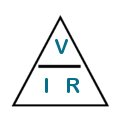If you wish to know what one quantity is in terms of the others simply cover that quantity.

#### Some Fundamental Equations

From the definition of Coulomb we can derive:

Q = I t

Where Q = charge in Coulombs,
I = current in Amps,
t = time in seconds,

Examples

If 3A of current flows through point A for 5seconds, how must charge passes through point A?

Using Q = I t
with I = 3, t = 5
we get Q = 15 C

Given that 18C of charge flow past a point B for 10 seconds, what is the average current through B?

Using Q = I t
Transposing,    I = Q/t
with Q = 18, t = 10
we get I = 1.8A

How long will it take to charge a capacitor to 15C if current flow to it is maintained at 3A?

Using Q = I t
Transposing,    t = Q/I
with Q = 15C, I = 3A
we get t = 5s